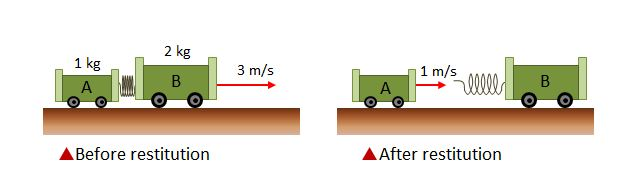# After restitutionTwo carts $A$ and $B$ connected by a compressed spring are moving at a constant speed of $3$ m/s on a frictionless floor as shown above left. At a certain moment, the compression of the spring is released, separating the two carts as shown above right. The masses of $A$ and $B$ are $1$ kg and $2$ kg, respectively, and the velocity of $A$ after the restitution of the spring is $1$ m/s. What is the velocity of $B$ after the restitution of the spring? (Ignore air resistance and the mass of the spring, and assume the direction of the red arrows is the positive(+) direction.)

×